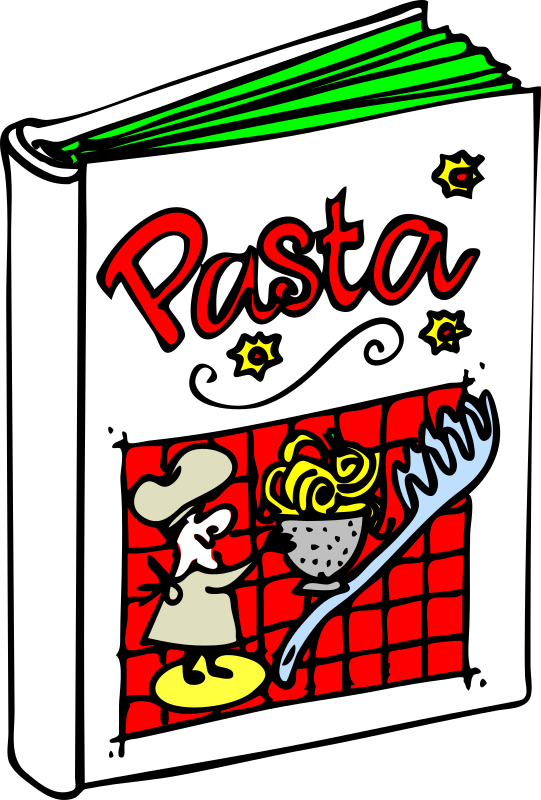Community Supported
Section Title Primary information Description and Notes Suggested resources
Lesson Length The expected lesson length will be approximately one hour

Some variation possible.

Assessment Level 1

Students The lesson is suitable for KS3 and KS4 students Since this is a new specification, the lesson is suitable for Year 7 to 9 students and differentiated by outcome
Overall Focus The lesson focus will be to introduce students to different ways that algorithms work This lesson will introduce the ways algorithms work so students can then compare their results

Lesson Links: 1, 2, 3, 4, 5, 6, 7, 8, 9, 10, 11, 12, 13, 14, 15, 17, 18, 19, 20, 21, 22, 23, 24, 25, 26, 27, 28, 29, 30, 31, 32, 33, 34, 35, 36, 37, 38, 39

Key words algorithm, abstraction, programming language, instruction, open source, digital, file types, variable These words need to be reinforced throughout the series of lessons
Assessment Identify different algorithms that target the same task Evidence here will be student's reflecting on what they understand and assessor observations
Key Questions Some questions to get learners thinking about the topics Looking at the way algorithms do what they are designed to do, but could it be better.What examples can they think of where different instructions will lead to the same result?  Can they think of why this might be useful?  What makes students decide on a particular "algorithm" such as going to school.  What factors do they consider, i.e avoid someone's house, good view, scary dog etc.
Learning Objectives
• To understand the range of algorithms that can be used
• To describe and explain, with examples, how different algorithms can be used for the same end result
• To understand how algorithms are decided and what factors influence this choice

Students need to be introduced to a range of algorithms that do the same tasks but in different ways.  In a way, software is a good example.  One spreadsheet software will get the same results as another, but might use a slightly different method.

If you are using the Learning Machine learning system, students can add their comments and material to this site as evidence of their growing understanding.  The site, if used consistently, will show progression via charts of activity and outcomes.
Teaching and Learning Elements
• Introduce some examples of algorithms in every day life and discuss their structure
• A teacher led demonstration of your view of some of these algorithms, as a guide to what they do and why
• Students can break into groups and work on their own ideas about the introduced algorithms
• Class discussion and evaluation of the wider uses of models and algorithms
• Summarize the key points and reinforce the importance of routines in good algorithm design

Students need to be taught some of the fundamentals of algorithms and how they can be constructed, but also some of the variation in them.  This will lead in to more quality programming from the students later on as they realise the best way to write routines (algorithms) for tasks.

Lesson Structure Possible structure
1. introduce some examples of algorithms
2. show students some more detailed examples of algorithms and discuss some of the elements that make them work, i.e. clear instructions
3. group work to work on some of their own
4. discuss what they have learned and what they can apply
5. volunteers to share their work and explain how they came to their final versions
6. highlight next week's focus and issue homeworkUse some examples of different routines that illustrate different ways to achieve the same goals.  Ty to show as wide a variety as possible.  For example, the following are algorithms: a recipe; tying your shoes; making a model; converting numbers (i.e percentages to fractions or Fahrenheit to Celsius); searching for homework references etc.

Plenty of examples onThis algorithm shows how diagnosis of a childhood illness is worked out.

The image shown here shows an algorithm for deciding to play or not.

The password generator we looked at in an earlier lesson also uses an algorithm to find the best password for users based on factors such as mixing capitals and small letters, numbers, symbols etc.

You can even make algorithms to herd sheep.

On the algorithm link in the key words box above, there is an example of various algorithms applied to sorting cards.  Students can sort them by numbers, colours, suits, style (number or picture cards) etc.

Show how common place algorithms are and how easy it is to get the same result with two different methods.

Homework Get students to write a 2 different recipes for making a pizza: one detailed, one simple Students can vary their homework depending on their level of understanding Get students to document their recipes on their portfolio system for assessment